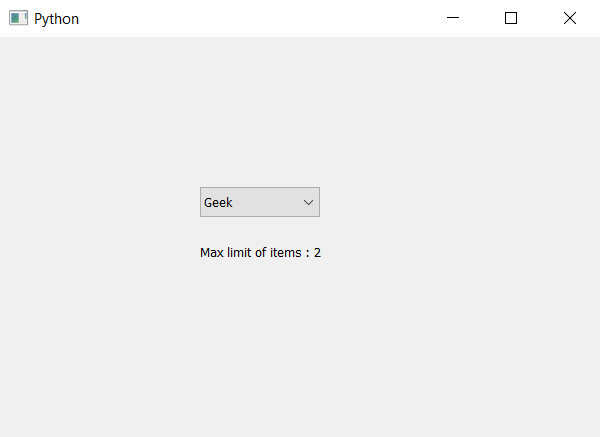# PyQt5 – How to know maximum number of items limit in ComboBox

In this article we will see how we can know maximum number limit of items in the combo box. When we create a combo box there is no limit set to the items we can add any number of items although sometimes condition arises to set the maximum limit to the items. In order to set the maximum limit to the number of items we use `setMaxCount` method.

In order to know the max limit we use `maxCount` method.

Syntax : combo_box.maxCount()

Argument : It takes no argument

Return : It returns integer

Below is the implementation –

 `# importing libraries ` `from` `PyQt5.QtWidgets ``import` `*`  `from` `PyQt5 ``import` `QtCore, QtGui ` `from` `PyQt5.QtGui ``import` `*`  `from` `PyQt5.QtCore ``import` `*`  `import` `sys ` ` `  ` `  `class` `Window(QMainWindow): ` ` `  `    ``def` `__init__(``self``): ` `        ``super``().__init__() ` ` `  `        ``# setting title ` `        ``self``.setWindowTitle(``"Python "``) ` ` `  `        ``# setting geometry ` `        ``self``.setGeometry(``100``, ``100``, ``600``, ``400``) ` ` `  `        ``# calling method ` `        ``self``.UiComponents() ` ` `  `        ``# showing all the widgets ` `        ``self``.show() ` ` `  `    ``# method for widgets ` `    ``def` `UiComponents(``self``): ` ` `  `        ``# creating a combo box widget ` `        ``self``.combo_box ``=` `QComboBox(``self``) ` ` `  `        ``# setting geometry of combo box ` `        ``self``.combo_box.setGeometry(``200``, ``150``, ``120``, ``30``) ` ` `  `        ``# geek list ` `        ``geek_list ``=` `[``"Geek"``, ``"Geeky Geek"``, ``"Legend Geek"``, ``"Ultra Legend Geek"``] ` ` `  `        ``# adding list of items to combo box ` `        ``self``.combo_box.addItems(geek_list) ` ` `  `        ``# setting maximum number limit of items ` `        ``self``.combo_box.setMaxCount(``2``) ` ` `  `        ``# getting max number limit ` `        ``limit ``=` `self``.combo_box.maxCount() ` ` `  `        ``# creating label to show the limit ` `        ``label ``=` `QLabel(``"Max limit of items : "` `+` `str``(limit), ``self``) ` ` `  `        ``# setting geometry of label ` `        ``label.setGeometry(``200``, ``200``, ``250``, ``30``) ` ` `  `# create pyqt5 app ` `App ``=` `QApplication(sys.argv) ` ` `  `# create the instance of our Window ` `window ``=` `Window() ` ` `  `# start the app ` `sys.exit(App.``exec``()) `

Output :My Personal Notes arrow_drop_upCheck out this Author's contributed articles.

If you like GeeksforGeeks and would like to contribute, you can also write an article using contribute.geeksforgeeks.org or mail your article to contribute@geeksforgeeks.org. See your article appearing on the GeeksforGeeks main page and help other Geeks.

Please Improve this article if you find anything incorrect by clicking on the "Improve Article" button below.

Article Tags :

Be the First to upvote.

Please write to us at contribute@geeksforgeeks.org to report any issue with the above content.